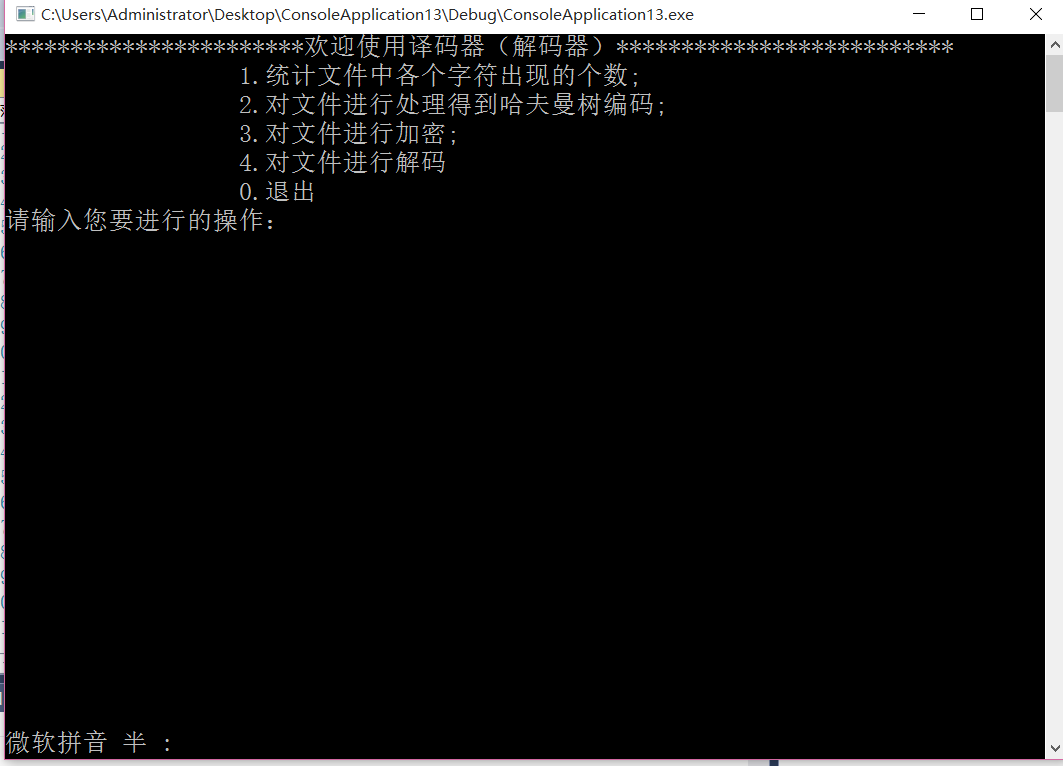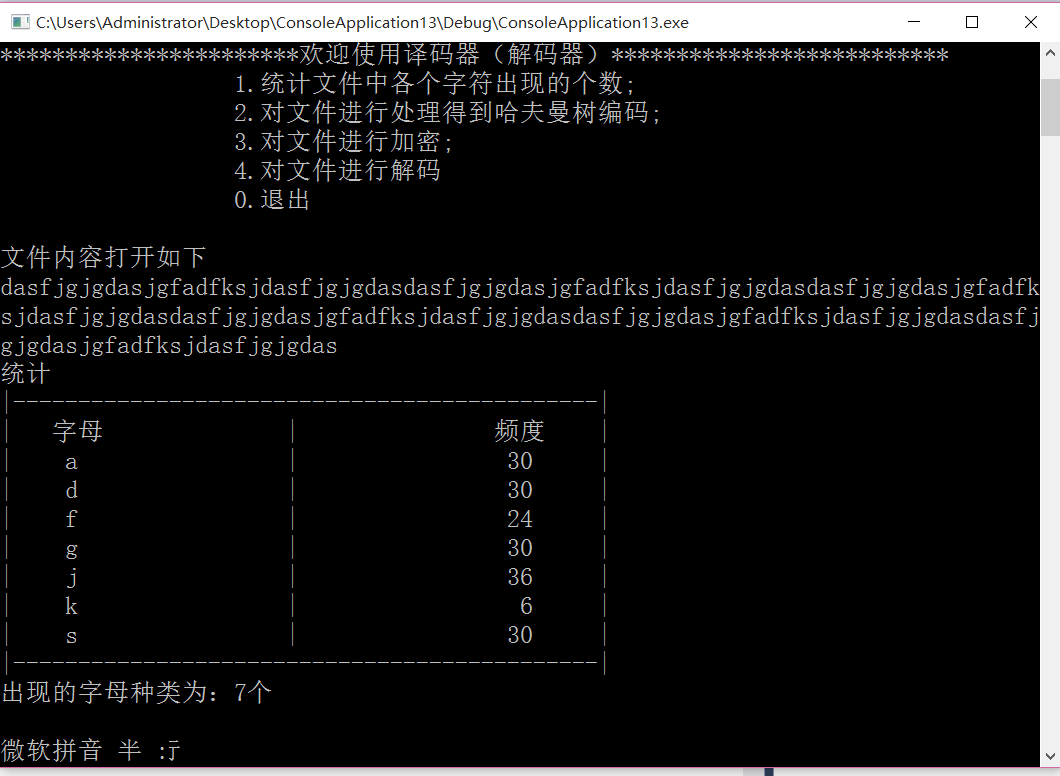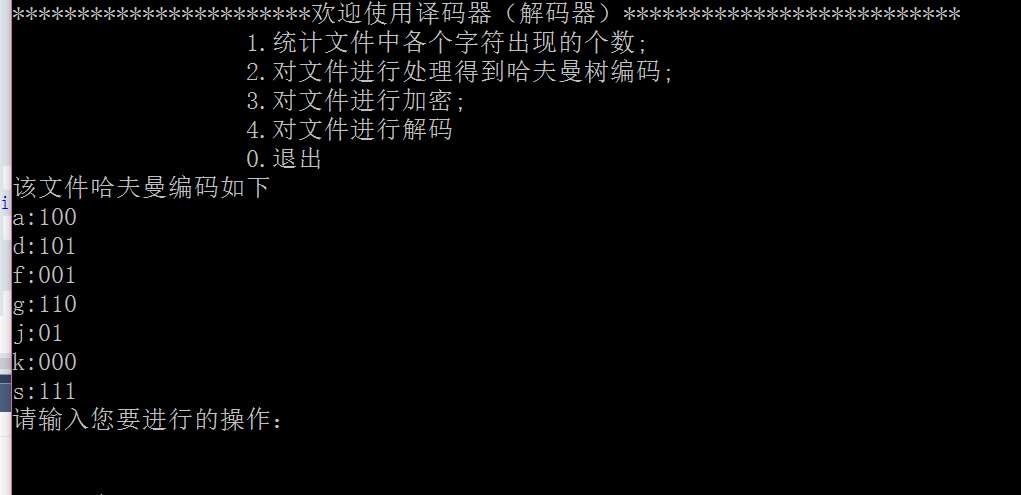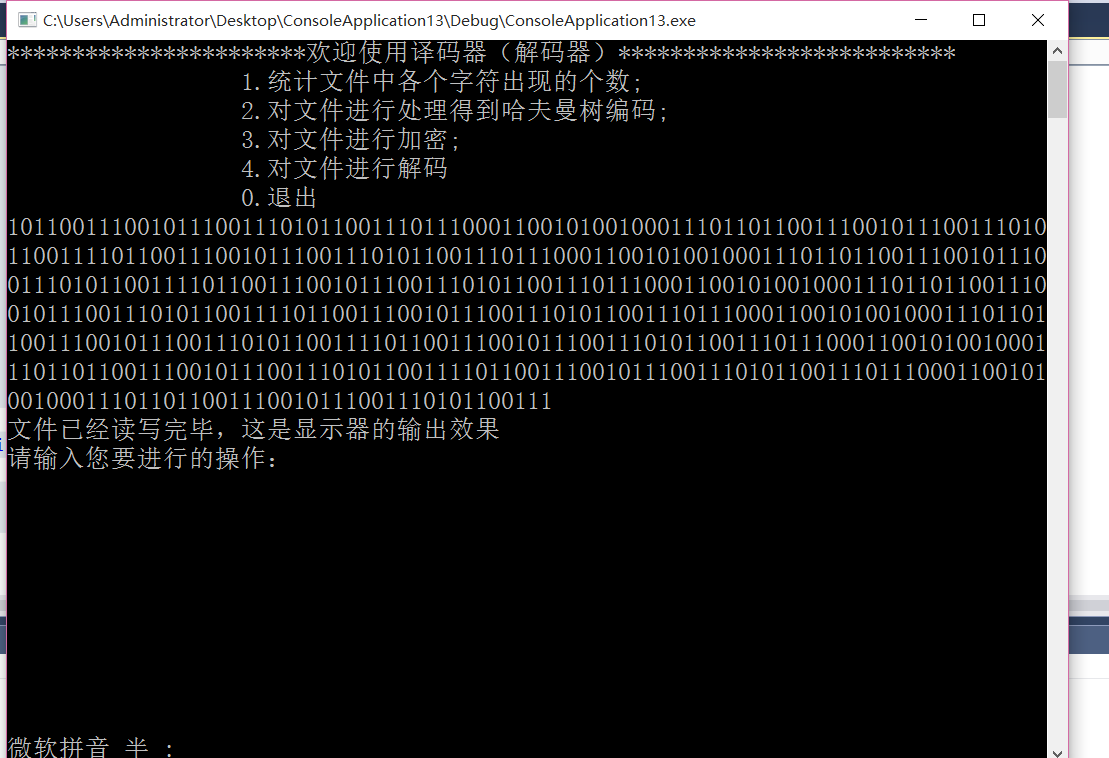• 数据结构哈夫曼树课程设计，完整课程设计，并附有全部代码。
• 用C++实现哈夫曼树的建立生成代码、可用Easy-X简单实现哈夫曼树的图像化、压缩包内附带了Easy-X安装包，运行代码前需安装Easy-X，并有相应课程设计报告，可用于数据结构、密码学、计算机网络等
• 数据结构课程设计报告设计题目：哈夫曼树应用专 业 ： 软件工程班 级 ： 软件学 生 ：学 号 ：指导教师 ： 罗作民 / 张翔起止时间 ：2011-07-04—2011-07-082011 年 春季 学期目 录一．具体任务…..21功能…………...
数据结构课程设计报告设计题目：哈夫曼树应用专     业 ：    软件工程班     级 ：    软件学     生 ：学     号 ：指导教师  ：  罗作民 / 张翔起止时间  ：2011-07-04—2011-07-082011 年  春季  学期目  录一．具体任务…..21功能……………………………………………………………………………...22分步实施………………………………………………………………………...2.3要求……………………………………………………………………………...2二．哈夫曼编码21问题描述22.基本要求33实现提示3三．设计流程图41建立哈夫曼树…………………………………………………………………...42编码……………………………………………………………………………...53译码……………………………………………………………………………...64主程序…………………………………………………………………………...7四．设计概要81问题哈夫曼的定义..............................................................................................8..2所实现的功能函数如下………………………………………………………..83功能模块………………………………………………………………………..8五．源程序9六．调试分析15七．心得与体会18八．参考文献18一、任务题目：哈夫曼树应用1.功能：1．从终端读入字符集大小n，以及n个字符和n个权值，建立哈夫曼树并将它存于文件hfmTree中.将已在内存中的哈夫曼树以直观的方式(比如树)显示在终端上；2．利用已经建好的哈夫曼树(如不在内存，则从文件htmTree中读入)，对文件ToBeTran中的正文进行编码，然后将结果存入文件CodeFile中，并输出结果，将文件CodeFile以紧凑格式先是在终端上，每行50个代码。同时将此字符形式的编码文件写入文件CodePrint中。3．利用已建好的哈夫曼树将文件CodeFile中的代码进行译码，结果存入文件TextFile中，并输出结果。2.分步实施：初步完成总体设计，搭好框架，确定人机对话的界面，确定函数个数；完成最低要求：完成功能1；进一步要求：完成功能2和3。有兴趣的同学可以自己扩充系统功能。3.要求：1)界面友好，函数功能要划分好2)总体设计应画一流程图3)程序要加必要的注释要提供程序测试方案程序一定要经得起测试，宁可功能少一些，也要能运行起来，不能运行的程序是没有价值的。二、哈夫曼编码1. 问题描述利用赫夫曼编码进行通信可以大大提高信道利用率，缩短信息传输时间，降低传输成本。这要求在发送端通过一个编码系统对待传输数据预先编码，在接收端将传来的数据进行译码(复原)。对于双工信道(即可以双向传输信息的信道)，每端都需要一个完整的编/译码系统。试为这样的信息收发站编写一个赫夫曼码的编/译码系统。基本要求一个完整的系统应具有以下功能：(1) I：初始化(Initialization)。从终端读入字符集大小n，以及n个字符和n个权值，建立赫夫曼树，并将它存于文件hfmTree中。(2) E：编码(Encoding)。利用已建好的赫夫曼树(如不在内存，则从文件hfmTree中读入)，对文件ToBeTran中的正文进行编码，然后将结果存入文件CodeFile中。(3) D：译码(Decoding)。利用已建好的赫夫曼树将文件CodeFile中的代码进行译码，结果存入文件Textfile中。实现提示(1) 编码结果以文本方式存储在文件Codefile中。(2) 用户界面可以设计为“菜单”方式：显示上述功能符号，再加上“Q”，表示退出运行Quit。请用户键入一个选择功能符。此功能执行完毕后再显示此菜单，直至某次用户选择了“Q”为止。(3) 在程序的一次执行过程中，第一次执行I， D或C命令之后，赫夫曼树已经在内存了，不必再读入。每次执行中不一定执行I命令，因为文件hfmTree可能早已建好。三、设计流程图
展开全文• 老师看过的，得分很高!有详细的代码和流程图！果断下载呀，亲！
• 将任意指定的文本文件中的字符统计后，按Huffman编码方式对文件进行编码，并保存码表及建立的Huffman；用给定的码表对用Huffman方式编码的文件进行压缩和解压缩。 总体设计 详细设计 测试结果
• >(1)初始化：键盘输入字符集大小n、n个字符和n个权值，建立哈夫曼树； (2)编码：利用建好的哈夫曼树生成哈夫曼编码； (3)输出编码； (4)设字符集及频度如下表： 字符 空格 A B C D E F G H I J K L M...
• 一、课程设计题目：哈夫曼树应用 二、课程设计要求： 1) 从终端读入字符集大小n，以及n个字符和n个权值，建立哈夫曼树并将它存于文件hfmTree中.将已在内存中的哈夫曼树以直观的方式（比如树）显示在终端上； 2) 利用...
• 一、课程设计题目：哈夫曼树应用 二、课程设计要求： 1) 从终端读入字符集大小n，以及n个字符和n个权值，建立哈夫曼树并将它存于文件hfmTree中.将已在内存中的哈夫曼树以直观的方式（比如树）显示在终端上； 2) 利用...
• 哈夫曼树C++设计，很做的，思维，代码都是一等的
• 哈夫曼树_数据结构课程设计《 数据结构 》课程设计——赫夫曼编码/译码器设计指导教师：孙树森、周维达班级：09数媒(2)班学号：姓名：林真超数据结构课程设计实验报告一、题目：赫夫曼编码/译码器设计二、目的：1、...
哈夫曼树_数据结构课程设计《 数据结构 》课程设计——赫夫曼编码/译码器设计指导教师：孙树森、周维达班级：09数媒(2)班学号：姓名：林真超数据结构课程设计实验报告一、题目：赫夫曼编码/译码器设计二、目的：1、提高分析问题、解决问题的能力，进一步巩固数据结构各种原理与方法。2、熟悉掌握一门计算机语言，可以进行数据算法的设计。三、要求3.1总体要求1、要充分认识课程设计对培养自己的重要性，认真做好设计前的各项准备工作。尤其是对编程软件的使用有基本的认识。2、既要虚心接受老师的指导，又要充分发挥主观能动性。结合课题，独立思考，努力钻研，勤于实践，勇于创新。3、独立按时完成规定的工作任务，不得弄虚作假，不准抄袭他人内容，否则成绩以不及格计。4、在设计过程中，要严格要求自己，树立严肃、严密、严谨的科学态度，必须按时、按质、按量完成课程设计。3.2实施要求1、理解赫夫曼编码/译码的确切意义。2、独立进行方案的制定，系统结构设计要合理。3、在程序开发时，则必须清楚主要实现函数的目的和作用，需要在程序书写时说明做适当的注释。在写课设报告时，必须要将主要函数的功能和参数做详细的说明。4、通过多组数据来检测该系统的稳定性和正确性。3.3 课程设计报告的内容及要求在完成课题验收后，学生应在规定的时间内完成课程设计报告一份(不少于2000字)。一、实验目的进一步掌握最优二叉树的含义。掌握最优二叉树的结构特征，以及各种存储结构的特点及使用范围。熟练掌握哈夫曼树的建立和哈夫曼编码方法。掌握用指针类型描述、访问和处理运算。二、实验原理哈夫曼(Huffman)编码属于码词长度可变的编码类，是哈夫曼在1952年提出的一种编码方法，即从下到上的编码方法。同其他码词长度可变的编码一样，可区别的不同码词的生成是基于不同符号出现的不同概率。生成哈夫曼编码算法基于一种称为“编码树”(coding tree)的技术。算法步骤如下：(1)初始化，根据符号概率的大小按由大到小顺序对符号进行排序。(2)把概率最小的两个符号组成一个新符号(节点)，即新符号的概率等于这两个符号概率之和。(3)重复第2步，直到形成一个符号为止(树)，其概率最后等于1。(4)从编码树的根开始回溯到原始的符号，并将每一下分枝赋值为1，上分枝赋值为0。译码的过程是分解电文中字符串，从根出发，按字符“0”或“1”确定找做孩子或右孩子，直至叶子节点，便求得该子串相应的字符。三、实验内容(一)需求分析一个完整的系统应具有以下功能：(1) I：初始化。从终端读入字符集大小n，以及n个字符和n个权值，建立哈夫曼树，并将它存于文件hfmTree中。(2) E：编码。利用已建好的哈夫曼树，对文件ToBeTran中的正文进行编码，然后将结果存入文件CodeFile中。(3) D：译码。利用已建好的哈夫曼树将文件CodeFile中的代码进行译码，结果存入文件Textfile中。(4) P：印代码文件(Print).将文件CodeFile以紧凑格式显示在终端上，每行50个代码。同时将此字符形式的编码文件写入文件CodePrin中。(5) T：印哈夫曼树(Treeprinting).将已在内存中的哈夫曼树以直观的方式(比如树)显示在终端上，同时将此字符形式的哈夫曼树写入文件TreePrint 中。(二)实验步骤1.定义结点结构，定义哈夫曼树结构；2.初始化哈夫曼树，存储哈夫曼树信息；3.定义求哈夫曼编码的函数；4.定义译哈夫曼编码的函数；5.写出主函数。6.测试系统。(三)概要设计1 设计思想赫夫曼树用邻接矩阵作为存储结构，借助静态链表来实现遍历。2 函数间的关系函数间的关系如图所示：图3.1 函数间的关系3 数据结构与算法设计赫夫曼编\译码器的主要功能是先建立赫夫曼树，然后利用建好的赫夫曼树生成赫夫曼编码后进行译码 。在数据通信中，经常需要将传送的文字转换成由二进制字符0、1组成的二进制串，称之为编码。构造一棵赫夫曼树，规定赫夫曼树中的左分之代表0，右分支代表1，则从根节点到每个叶子节点所经过的路径分支组成的0和1的序列便为该节点对应字符的编码，称之为赫夫曼编码。最简单的二进制编码方式是等长编码。若采用不等长编码，让出现频率高的字符具有较短的编码，让出现频率低的字符具有较长的编码，这样可能缩短传送电文的总长度。赫夫曼树课用于构造使电文的编码总长最短的编码方案。其主要流程图如图3.2所示。图3.2 赫夫曼树编\译码器流程图4.功能函数模块划分void main()void printhead()void printree(HuffmanTree HT,int w) //打印赫夫
展开全文• 数据结构课程设计哈夫曼树。 自己修改后的完整版。 简单的c语言做的
• 哈夫曼编码课程设计,我要让所以人都知道写一个哈夫曼编码便不是难事。
• ## 哈夫曼树的课程设计

千次阅读 热门讨论 2009-12-27 09:59:00
我们利用哈夫曼算法建立一棵哈夫曼树(最优二叉树)，同时将数据出现的频率作为权值赋给哈夫曼树中的结点。根据建立好的哈夫曼树我们进行编码，从根结点出发在左子树则标为0，右则标为1。直到到指定的叶子结点，然后将...
摘  要
在计算机信息处理中，“哈夫曼编码”是一种一致性编码法（又称"熵编码法"），用于数据的无损耗压缩。我们会发现一些数据经常出现的频率高，有些出现的频率低。我们利用哈夫曼算法建立一棵哈夫曼树(最优二叉树)，同时将数据出现的频率作为权值赋给哈夫曼树中的结点。根据建立好的哈夫曼树我们进行编码，从根结点出发在左子树则标为0，右则标为1。直到到指定的叶子结点，然后将遍历过程中标记的0，1代码存在一个数组中。以此实现将使用频率高的字符的编码尽可能的少，也就使得总的长度减少。在哈夫曼编码的基础上进行解码，就可以还原压缩的数据。
Abstract In the computer information processing, "Huffman coding" is a consistent coding method (also known as "entropy coding method") for lossless data compression. We will find some of the data often appear in high frequency, some of the frequency of the low. Huffman algorithm we use to establish a Huffman tree (the optimal binary tree), while the frequency of the data as the weights assigned to Huffman tree nodes. According to our well-established Huffman coding, starting from the root node in the left subtree is marked as 0, the right is labeled 1. Up to the designated leaf nodes, and then traverse the process of marking the existence of a 0,1 code array. Implementation will be used as the character encoding of high frequency low as possible, thus making the total length of the reduction. In the Huffman coding based on the decoding, you can restore the compressed data.

目  录

第一章    课程设计目的
第二章   课程设计的思路
2.1  哈夫曼树的建立
2.2  哈夫曼编码
2.3  哈夫曼解码
第三章    详细设计
3.1 模块分块
3.2 详细代码
第四章    测试结果
4.1  创建哈夫曼树
4.2 哈夫曼编码
4.3 哈夫曼解码
4.4 退出
第五章  总结
5.1   创建哈夫曼树
5.2    哈夫曼编码
5.5   哈夫曼解码
5.4    程序运行中遇到的问题
5.5  感想
第六章   参考资料

第一章    课程设计目的
1、掌握哈夫曼树的建立。
2、掌握哈夫曼的编码和解码。
3、加深对树的理解以及树的实际应用。
第二章   课程设计的思路
2.1  哈夫曼树的建立
前提：对给定的n个结点的权值和字符。
目标：建立一棵哈夫曼树。
算法输入：n个结点的权值和字符。
算法输出：哈夫曼树。
算法思想：
<1>哈夫曼树的存储结构：用哈夫曼算法得的哈夫曼树中共有2n-1个结点，其中n个叶结点是初始森林中的n个孤立根结点，其余n-1结点是构造过程中产生的度为2的结点。故只需定义一个哈夫曼树数组开辟2n-1个空间来存储哈夫曼树。为了与哈夫曼编码相匹配我们需要定义5域：权值域、左孩子域、右孩子域、双亲�
展开全文算法 tree processing character algorithm
• ## 哈夫曼树的应用——课程设计

千次阅读 多人点赞 2016-12-26 13:20:18
这个是我去年的课程设计报告，因为有学弟要我就整理了下，功能很简单，就一个加密解密还有求哈夫曼编码，但足够满足老师的要求，现在传给有需要的童鞋参考~ 完整的代码及报告 以下是实验报告内容    哈夫曼树...
这个是我去年的课程设计报告，因为有学弟要我就整理了下，功能很简单，就一个加密解密还有求哈夫曼编码，但足够满足老师的要求，现在传给有需要的童鞋参考~

完整的代码及报告

以下是实验报告内容

哈夫曼树的应用——对文件进行解码和译码
一、       需求分析说明
随着信息时代的到来，信息越来越多，如何压缩信息已经是重要课题。特别是多媒体技术的发展，使得信息量大的问题凸显。
哈夫曼编码(Huffman Coding)是一种无损压缩编码方式，以哈夫曼树—即最优二叉树，带权路径长度最小的二叉树，经常应用于数据压缩。在计算机信息处理中，“哈夫曼编码”是一种一致性编码法（又称"熵编码法"），用于数据的无损耗压缩。这一术语是指使用一张特殊的编码表将源字符（例如某文件中的一个符号）进行编码。这张编码表的特殊之处在于，它是根据每一个源字符出现的估算概率而建立起来的（出现概率高的字符使用较短的编码，反之出现概率低的则使用较长的编码，这便使编码之后的字符串的平均期望长度降低，从而达到无损压缩数据的目的）。这种方法是由David.A.Huffman发展起来的。例如，在英文中，e的出现概率很高，而z的出现概率则最低。当利用哈夫曼编码对一篇英文进行压缩时，e极有可能用一个位(bit)来表示，而z则可能花去
25个位（不是26）。用普通的表示方法时，每个英文字母均占用一个字节（byte），即8个位。二者相比，e使用了一般编码的1/8的长度，z则使用了 3倍多。倘若我们能实现对于英文中各个字母出现概率的较准确的估算，就可以大幅度提高无损压缩的比例。
当然本软件的目的不在于如何去压缩一个文件信息，而是通过建立哈夫曼树，由哈夫曼树编得二进制码，再译码，来了解哈夫曼树编码实现过程。(庞大的翻译内容会进行报错)

二、       主要功能
1.统计文件中各个字符出现的个数;
2.对文件进行处理得到哈夫曼树编码;
3.对文件进行加密;
4.对文件进行解码
三、       所运用到的知识点
（1）链式存储结构（用来从文件中读取数据，构造哈夫曼编码）
（2）队列的应用（主要用在解码那一块，从文件中逐个读取字符，进入队列，再一个一个判断是否是哪个编码值）
（3）构造最优二叉树，对树的基本操作
（4）对文件的操作（打开文件，读写文件）
四、核心代码实现
（一）   菜单块
while (i != 0)
{
cout << "***********************欢迎使用译码器（解码器）**************************" << endl;
cout << "                  1.统计文件中各个字符出现的个数;                        " << endl;
cout << "                  2.对文件进行处理得到哈夫曼树编码;                      " << endl;
cout << "                  3.对文件进行加密;                                      " << endl;
cout << "                  4.对文件进行解码                                       " << endl;
cout << "                  0.退出                                                 " << endl;
switch (i)
{
case 1:
List.creatlist();
List.disp();
List.total(); break;
case 2:
L.CreateHT();
L.CreateHCode();
L.DispHCode(); break;
case 3:
L.CreateHT();
L.CreateHCode();
L.Revertext(); break;
/*	case 0:
exit(0);*/
case 4:   /*将二进制转化为字母*/
L.CreateHT();
L.CreateHCode();
L.decode(); break;
case 0:
cout << "欢迎下次使用译码器（解码器）" << endl;
break;
}
cout << "请输入您要进行的操作：";
cin >> i;
system("cls");
}

（二）   统计字符
void total()                           //利用一个数组，统计字母出现次数
{
int j = 0;
int count = { 0 };             //设置初始化
int num;
while (s)
{
num = s->data;
if (num != 32)               //不计算空格出现的频度
{
count[num - 0]++;
}
s = s->next;
}
cout << "统计" << endl;
cout << "|---------------------------------------------|" << endl;
cout << "|   字母              |               频度    |" << endl;
for (int i = 0; i < 127; i++)
{
if (count[i])
{
aa[j].data = i;
aa[j].weight = count[i];
j++;
cout << "|    " << char(i) << "                |            " << setw(6) << count[i] << "     |" << endl;
}
}
Count = j;
cout << "|---------------------------------------------|" << endl;
cout << "出现的字母种类为：" << Count << "个" << endl;
cout << endl;

}
};
（三）   创建哈夫曼树
void HuffmanClass<T>::CreateHT()               //构造哈夫曼树
{
int i, k, lnode, rnode;
double min1, min2;
for (i = 0; i < (2 * no - 1); i++)                    //所有结点的相关域置初值-1
{
ht[i].parent = -1;
ht[i].lchild = -1;
ht[i].rchild = -1;
}
for (i = no; i < (2 * no - 1); i++)                  //构造哈夫曼树，仅求非叶子结点
{
min1 = min2 = 32767.0;                          //初始时置最大权值
lnode = rnode = -1;                             //lnode和rnode为两个权重最小的节点位置
for (k = 0; k <= (i - 1); k++)                   //在ht数组中找权值最小的两个结点

if (ht[k].parent == -1)                      //只在二叉树的根节点中寻找
{
if (ht[k].weight < min1)
{
min2 = min1;
rnode = lnode;
min1 = ht[k].weight;
lnode = k;
}
else if (ht[k].weight < min2)
{
min2 = ht[k].weight;
rnode = k;
}
}

ht[lnode].parent = i;                   //把后面的no-1个非叶子结点处理完毕
ht[rnode].parent = i;
ht[i].weight = ht[lnode].weight + ht[rnode].weight;
ht[i].lchild = lnode;                                 //ht[i]作为双亲结点
ht[i].rchild = rnode;
}
}
（四）   得到哈夫曼编码
void HuffmanClass<T>::CreateHCode()                       //根据哈夫曼树求哈夫曼编码
{
int i, f, c;
for (i = 0; i < no; i++)                            //遍历下标从0到no-1的叶子结点
{
hcd[i].start = no;                              //从hcd[i].cd[no]开始放置哈夫曼编码
c = i;
f = ht[i].parent;                               //找其双亲结点
while (f != -1)                                 //循环直至无双亲结点即到达树根结点
{
if (ht[f].lchild == c)                       //当前结点是双亲结点的左孩子结点
{
hcd[i].cd[hcd[i].start] = '0';
/*cout << hcd[i].cd[hcd[i].start]<<endl;*/
}
else
{
hcd[i].cd[hcd[i].start] = '1';
}//当前结点是双亲结点的右孩子结点

hcd[i].start--;
c = f;
f = ht[f].parent;                        //再对双亲进行同样的操作
}
hcd[i].start++;                   //start指向哈夫曼编码最开始字符
}
}
（五）   对文件进行加密
void  HuffmanClass<T>::Revertext()
{
SqQueueClass<char> L;
L.initQueue();
FILE *fin, *fout;
char ch;
if ((fin = fopen("D:\\test.txt", "r")) == NULL)
{
cout << "无法打开此文件" << endl;
exit(0);
}
if ((fout = fopen("D:\\tex.txt", "w")) == NULL)
{
cout << "无法打开此文件" << endl;
exit(0);
}
ch = fgetc(fin);//一个一个读出来
while (!feof(fin))
{
for (int i = 0; i < no; i++)
{
if (ch == ht[i].data)
{
for (int j = hcd[i].start; j <= no; j++)
{
cout << hcd[i].cd[j];
fputc(hcd[i].cd[j], fout);
}
}
}
ch = fgetc(fin);  //从fin 文件中打开,再次读
}
cout << endl << "文件已经读写完毕，这是显示器的输出效果" << endl;
fclose(fin);
fclose(fout);
}
（六）   对文件进行解码
void HuffmanClass<T>::decode()//解码
{
cout << "文件解码为:" << endl;
int mark = 0;//设置标志
int q, j, ii, jj, k, tt;
int kk = 0;
char temp[MaxQueue][MaxQueue];
char temp1[MaxQueue][MaxQueue];
SqQueueClass<char> L;
L.initQueue();
FILE *fin;
char ch;
if ((fin = fopen("D:\\test1.txt", "r")) == NULL)
{
cout << "无法打开此文件" << endl;
exit(0);
}
for (int aa = 0; aa < no; aa++)
{
for (int bb = hcd[aa].start; bb <= no; bb++)
{
temp1[aa][(bb - hcd[aa].start)] = hcd[aa].cd[bb];
/*cout << temp1[aa][(bb - hcd[aa].start)] << " ";*/    /*保留*/
}
}
ch = fgetc(fin);//一个一个读出来
while (!feof(fin))
{
mark = 0;
L.enQueue(ch);//进队

for (j = 0, q = L.getfront() + 1; q != L.getrear() + 1; j++, q++)    //一个个获得临时的01(temp)序列，以便和哈夫曼编码比较
{
temp[kk][j] = L.data[q];
}
for (ii = 0; ii < no; ii++)//哈弗曼编码放到二维数组里了
{
int zz;
zz = 0;
for (jj = 0; jj <= no - hcd[ii].start; jj++)
{
if (temp[kk][jj] == temp1[ii][jj])//若两个相等
{
zz = zz + 1;
}

}
if (zz == no - hcd[ii].start + 1)
{
cout << ht[ii].data;
mark = 1; break;
}

}
if (mark == 1)
{
L.destory();//清空队列
/*cout << "清空" << endl;*/
}
ch = fgetc(fin);  //从fin 文件中打开,再次读
kk = kk + 1;
}
if (L.getfront() != L.getrear())
{
cout << "\n以下二进制串输入有错误：";
for (int j = L.getfront() + 1; j != L.getrear() + 1; j++)
cout << L.data[j];
}
else
cout << endl;
cout << endl << "文件已经读写完毕，这是显示器的输出效果" << endl;
fclose(fin);
}
五、       结果展示
菜单：计算字符出现的频度：得到哈夫曼编码：加密：解码：六、       总结
做这个课程设计是熬了两个晚上做出来的，其中有一个如何解密，如何判断从文件读取的数据构成的数组与我的每一个哈夫曼编码值是否相等，因为哈夫曼编码的存储方式是存在数组，且从start元素到no元素才是正确的值，所以非常麻烦。我一直在不停地用循环判断，甚至要重新写我如何得到哈夫曼的编码，因为实在太困难了。索性，学到了一个好方法，就是中断，通过中断可以查看自己的程序走到哪一步了，再跟着步骤和本应该有的步骤进行对比，就很容易发现错误，这一次最大的收获就是学会熟练使用中断。还有可以用到之前学到的知识点，比如这里用了队列进行读取数据，以前从没有想过队列可以这样用。还有要集思广益，不一定去网上找代码就是不好的，因为适合别人的代码不一定适合自己，还是要自己写，主要是学习他们的思路，然后用自己的方法做出来。这个.cpp的功能其实还有缺陷，就是如果我对非常多的‘0’‘1’进行译码是会出现错误，我不知道是什么问题，不过会继续深入，另外还有一个可以提升的地方是对文件的操作应该是让用户自己选择文件而不是固定文件，不过，因为自己的代码太冗余，很难实现这个操作，不过会继续学习如何写出漂亮的代码。

完整的代码：
#include<iostream>
#include<fstream>
#include<iomanip>
#include<string>
using namespace std;
#include<stdio.h>
#include<stdlib.h>
#define MaxSize 100
#define MaxQueue 100
{
char data;
};
struct pindu                                           //每个字母出现的频度结点类型
{
char data;         //字母
int weight;        //权值
};
template <typename T>
struct HTNode                                          //哈夫曼结点类型
{
T data;//结点值
double weight;//权值
int parent;//双亲结点
int lchild;//左孩子结点
int rchild;//右孩子结点
};

struct HCode                                           //哈夫曼编码类型
{
char cd[MaxSize];              //存放当前结点的哈夫曼编码
int start;                    //用cd[start..no]存放哈夫曼编码,包括start和no
};
class sqlist
{
private:
int Count;                          //计算个数
public:
pindu aa;                      //存放频度的信息
sqlist()
{
Count = 0;
}
void destorysqlist()                     //删除单链表，不能用析构，因为随时不用，会对哈夫曼数的构造造成影响
{
p = pre->next;
while (p != NULL)
{
delete pre;
pre = p; p = p->next;
}
delete pre;
}
int getCount()                       //得到权值个数
{
int j = 0;
int count = { 0 };             //设置初始化
int num;
while (s)
{
num = s->data;
if (num != 32)               //不计算空格出现的频度
{
count[num - 0]++;
}
s = s->next;
}
for (int i = 0; i < 127; i++)
{
if (count[i])
{
aa[j].data = i;
aa[j].weight = count[i];
j++;
}
}
Count = j;
return Count;
}
void creatlist()                     //创建单链表，把文件中的字母写进单链表中
{
FILE *fin;
char ch;
/*char filename = { 0 };
cout << "请输入文件路径：";
cin >> filename;*/
if ((fin = fopen("H:\\test.txt", "r")) == NULL)
{
cout << "无法打开此文件" << endl;
exit(0);
}
ch = fgetc(fin);
while (!feof(fin))
{
s->data = ch;
r->next = s;
r = s;
ch = fgetc(fin);  //从fin 文件中打开
}
r->next = NULL;
fclose(fin);
/*	cout << endl;
cout << endl;*/
}
void disp()                                 //输出单链表内容，即文章内容
{
cout << endl << "文件内容打开如下" << endl;
int count = { 0 };
while (s)
{
cout << s->data;
s = s->next;
}
cout << endl;
}
void total()                           //利用一个数组，统计字母出现次数
{
int j = 0;
int count = { 0 };             //设置初始化
int num;
while (s)
{
num = s->data;
if (num != 32)               //不计算空格出现的频度
{
count[num - 0]++;
}
s = s->next;
}
cout << "统计" << endl;
cout << "|---------------------------------------------|" << endl;
cout << "|   字母              |               频度    |" << endl;
for (int i = 0; i < 127; i++)
{
if (count[i])
{
aa[j].data = i;
aa[j].weight = count[i];
j++;
cout << "|    " << char(i) << "                |            " << setw(6) << count[i] << "     |" << endl;
}
}
Count = j;
cout << "|---------------------------------------------|" << endl;
cout << "出现的字母种类为：" << Count << "个" << endl;
cout << endl;

}
};

template <typename T>
class SqQueueClass                                        //队列进行解码操作
{
private:
int front;
int rear;
public:
T data[MaxQueue];
void initQueue()//初始化
{
front = rear = 0;
}
void destory()//清空队列
{
initQueue();
}
void enQueue(T e)//进队
{
//if ((rear + 1) % MaxQueue == front)//队满
//	return false;
rear = (rear + 1) % MaxQueue;
data[rear] = e;
}
void deQueue(T &e)//出队
{
/*if (rear == front)
return false;*/
front = (front + 1) % MaxQueue;
e = data[front];
}
void disp()
{
for (int i = front + 1; i <= rear; i++)
{
cout << data[i] << " ";
}
}
int getfront()
{
return front;
}
int getrear()
{
return rear;
}
};

template <typename T>
class HuffmanClass
{
private:
int no;                            //权值个数
HTNode<T> ht[MaxSize];              //存放哈夫曼树
HCode hcd[MaxSize];                 //存放哈夫曼编码
public:
HuffmanClass();					            //Setvalue设置初值
void CreateHT();                             //构造哈夫曼树
void CreateHCode();                          //根据哈夫曼树求哈夫曼编码
void DispHCode();                            //输出哈夫曼编码
void Revertext();                            //将文本转化成二进制代码存入另一个文件中
void decode();                                  //解码，把二进制转化成文字
};
template <typename T>
HuffmanClass<T>::HuffmanClass()                   //设置初值
{
pindu str;
sqlist List;//
List.creatlist();//
for (int i = 0; i < List.getCount(); i++)
{
str[i].data = List.aa[i].data;

str[i].weight = List.aa[i].weight;
}
no = List.getCount();    //权值个数
for (int i = 0; i <(2 * no - 1); i++)
{
ht[i].data = str[i].data;
ht[i].weight = str[i].weight;
}
}
template <typename T>

void HuffmanClass<T>::CreateHT()               //构造哈夫曼树
{
int i, k, lnode, rnode;
double min1, min2;
for (i = 0; i < (2 * no - 1); i++)                    //所有结点的相关域置初值-1
{
ht[i].parent = -1;
ht[i].lchild = -1;
ht[i].rchild = -1;
}
for (i = no; i < (2 * no - 1); i++)                  //构造哈夫曼树，仅求非叶子结点
{
min1 = min2 = 32767.0;                          //初始时置最大权值
lnode = rnode = -1;                             //lnode和rnode为两个权重最小的节点位置
for (k = 0; k <= (i - 1); k++)                   //在ht数组中找权值最小的两个结点

if (ht[k].parent == -1)                      //只在二叉树的根节点中寻找
{
if (ht[k].weight < min1)
{
min2 = min1;
rnode = lnode;
min1 = ht[k].weight;
lnode = k;
}
else if (ht[k].weight < min2)
{
min2 = ht[k].weight;
rnode = k;
}
}

ht[lnode].parent = i;                   //把后面的no-1个非叶子结点处理完毕
ht[rnode].parent = i;
ht[i].weight = ht[lnode].weight + ht[rnode].weight;
ht[i].lchild = lnode;                                 //ht[i]作为双亲结点
ht[i].rchild = rnode;
}
}
template <typename T>
void HuffmanClass<T>::CreateHCode()                       //根据哈夫曼树求哈夫曼编码
{
int i, f, c;
for (i = 0; i < no; i++)                            //遍历下标从0到no-1的叶子结点
{
hcd[i].start = no;                              //从hcd[i].cd[no]开始放置哈夫曼编码
c = i;
f = ht[i].parent;                               //找其双亲结点
while (f != -1)                                 //循环直至无双亲结点即到达树根结点
{
if (ht[f].lchild == c)                       //当前结点是双亲结点的左孩子结点
{
hcd[i].cd[hcd[i].start] = '0';
/*cout << hcd[i].cd[hcd[i].start]<<endl;*/
}
else
{
hcd[i].cd[hcd[i].start] = '1';
}//当前结点是双亲结点的右孩子结点

hcd[i].start--;
c = f;
f = ht[f].parent;                        //再对双亲进行同样的操作
}
hcd[i].start++;                   //start指向哈夫曼编码最开始字符
}
}
template <typename T>
void HuffmanClass<T>::DispHCode()
{
cout << "该文件哈夫曼编码如下" << endl;
for (int i = 0; i < no; i++)
{
cout << ht[i].data << ":";
for (int j = hcd[i].start; j <= no; j++)                 //因为start一直在变化，一直在减小，存的值是从下往上走的，就是start减小的顺序
{
cout << hcd[i].cd[j];
}
cout << endl;
}
}

template <typename T>
void  HuffmanClass<T>::Revertext()
{
SqQueueClass<char> L;
L.initQueue();
FILE *fin, *fout;
char ch;
if ((fin = fopen("H:\\test.txt", "r")) == NULL)
{
cout << "无法打开此文件" << endl;
exit(0);
}
if ((fout = fopen("H:\\tex.txt", "w")) == NULL)
{
cout << "无法打开此文件" << endl;
exit(0);
}
ch = fgetc(fin);//一个一个读出来
while (!feof(fin))
{
for (int i = 0; i < no; i++)
{
if (ch == ht[i].data)
{
for (int j = hcd[i].start; j <= no; j++)
{
cout << hcd[i].cd[j];
fputc(hcd[i].cd[j], fout);
}
}
}
ch = fgetc(fin);  //从fin 文件中打开,再次读
}
cout << endl << "文件已经读写完毕，这是显示器的输出效果" << endl;
fclose(fin);
fclose(fout);
}

template <typename T>
void HuffmanClass<T>::decode()//解码
{
cout << "文件解码为:" << endl;
int mark = 0;//设置标志
int q, j, ii, jj, k, tt;
int kk = 0;
char temp[MaxQueue][MaxQueue];
char temp1[MaxQueue][MaxQueue];
SqQueueClass<char> L;
L.initQueue();
FILE *fin;
char ch;
if ((fin = fopen("H:\\test1.txt", "r")) == NULL)
{
cout << "无法打开此文件" << endl;
exit(0);
}
for (int aa = 0; aa < no; aa++)
{
for (int bb = hcd[aa].start; bb <= no; bb++)
{
temp1[aa][(bb - hcd[aa].start)] = hcd[aa].cd[bb];
/*cout << temp1[aa][(bb - hcd[aa].start)] << " ";*/    /*保留*/
}
}
ch = fgetc(fin);//一个一个读出来
while (!feof(fin))
{
mark = 0;
L.enQueue(ch);//进队

for (j = 0, q = L.getfront() + 1; q != L.getrear() + 1; j++, q++)    //一个个获得临时的01(temp)序列，以便和哈夫曼编码比较
{
temp[kk][j] = L.data[q];
}
for (ii = 0; ii < no; ii++)//哈弗曼编码放到二维数组里了
{
int zz;
zz = 0;
for (jj = 0; jj <= no - hcd[ii].start; jj++)
{
if (temp[kk][jj] == temp1[ii][jj])//若两个相等
{
zz = zz + 1;
}

}
if (zz == no - hcd[ii].start + 1)
{
cout << ht[ii].data;
mark = 1; break;
}

}
if (mark == 1)
{
L.destory();//清空队列
/*cout << "清空" << endl;*/
}
ch = fgetc(fin);  //从fin 文件中打开,再次读
kk = kk + 1;
}
if (L.getfront() != L.getrear())
{
cout << "\n以下二进制串输入有错误：";
for (int j = L.getfront() + 1; j != L.getrear() + 1; j++)
cout << L.data[j];
}
else
cout << endl;
cout << endl << "文件已经读写完毕，这是显示器的输出效果" << endl;
fclose(fin);
}

{
HuffmanClass <char>L;
sqlist List;
int i;
cout << "***********************欢迎使用译码器（解码器）**************************" << endl;
cout << "                  1.统计文件中各个字符出现的个数;                        " << endl;
cout << "                  2.对文件进行处理得到哈夫曼树编码;                      " << endl;
cout << "                  3.对文件进行加密;                                      " << endl;
cout << "                  4.对文件进行解码                                       " << endl;
cout << "                  0.退出                                                 " << endl;
cout << "请输入您要进行的操作：";
cin >> i;
while (i != 0)
{
cout << "***********************欢迎使用译码器（解码器）**************************" << endl;
cout << "                  1.统计文件中各个字符出现的个数;                        " << endl;
cout << "                  2.对文件进行处理得到哈夫曼树编码;                      " << endl;
cout << "                  3.对文件进行加密;                                      " << endl;
cout << "                  4.对文件进行解码                                       " << endl;
cout << "                  0.退出                                                 " << endl;
switch (i)
{
case 1:
List.creatlist();
List.disp();
List.total(); break;
case 2:
L.CreateHT();
L.CreateHCode();
L.DispHCode(); break;
case 3:
L.CreateHT();
L.CreateHCode();
L.Revertext(); break;
/*	case 0:
exit(0);*/
case 4:   /*将二进制转化为字母*/
L.CreateHT();
L.CreateHCode();
L.decode(); break;
case 0:
cout << "欢迎下次使用译码器（解码器）" << endl;
break;
}
cout << "请输入您要进行的操作：";
cin >> i;
system("cls");
}
}
int main()
{
system("pause");
return 0;
}

代码注意事项：

软件：DEV C++
主要功能
1.统计文件中各个字符出现的个数;
2.对文件进行处理得到哈夫曼树编码;
3.对文件进行加密;
4.对文件进行解码
1.在H盘上创建一个名为test.txt的文件，存放a-z任意字符；
2.在H盘创建一个名为test1.txt的文件，存放01字符；
3.在H盘创建一个名为tex.txt的文件（可以什么都不放）；
（可自行换盘，代码要改）
功能一：求test.txt的各个字符出现的频度；
功能二：求出哈夫曼编码（根据test.txt中的字符）；
功能三：对test.txt的文件进行加密（根据求得的哈夫曼编码来），然后把结果存放到tex.txt中；
功能四：是对test1中的01字符进行解码，翻译成对应的字符，将结果输出到屏幕；（就是根据功能二得到的哈夫曼树，对test1.txt的内容进行解码）


展开全文• 这是一个数据结构相关的课程设计，主要是哈夫曼树方面的，对初学者有一定帮助
• 大学期间数据结构课程的一个实验，好像记得是课程设计来着！
• java课程设计关于哈夫曼树编码译码,输入一串字符串,将其根据字符出现的频率建哈夫曼树并根据树进行译码。 此word的代码完全拷贝在eclipse可直接运行,此文档仅供参考,希望各位能做出自己的修改成适合自己的文档。
• 建立哈夫曼树 编码 译码 详细的步骤 程序和流程图 心得体会等
• 计算哈夫曼树的WPL值 根据给定的n个权值（非负值），计算所构造哈夫曼树的WPL值。 基本要求： （1）根据给定的数据，建立哈夫曼树； （2）输出每个叶子结点的带权路径长度； （3）输出哈夫曼树的WPL值。 ...
• #include&lt;stdio.h&gt; #include&...#define M 2*N-1 //中结点总数 #include&lt;iostream&gt; using namespace std; typedef struct { char data;//结点值 int weight; /...C++
• 设计内容： 欲发一封内容为AABBCAB ……(共长 100 字符,其中:A 、B 、C 、D 、E 、F分别有7 、9 、12 、22 、23、27个)的电报报文,实现哈夫曼编码。
• 哈夫曼树和编码应用数据结构课程设计 任务和功能： （1）从终端读入字符集大小n，以及n个字符和n个权值，建立哈夫曼树的存储结构； （2）利用已经建好的哈夫曼树（如不在内存，则从文件htmTree中读入），对给定的n个...
• 课程设计(论文)任务书;- 1 -;- 1 -;一 设计任务 问题描述 设计一个利用哈夫曼算法的编码系统重复地显示并处理以下项目 直到选择退出为止 基本要求 初始化键盘输入字符集大小 nn 个字符和 n 个权值建立哈夫 曼编码......